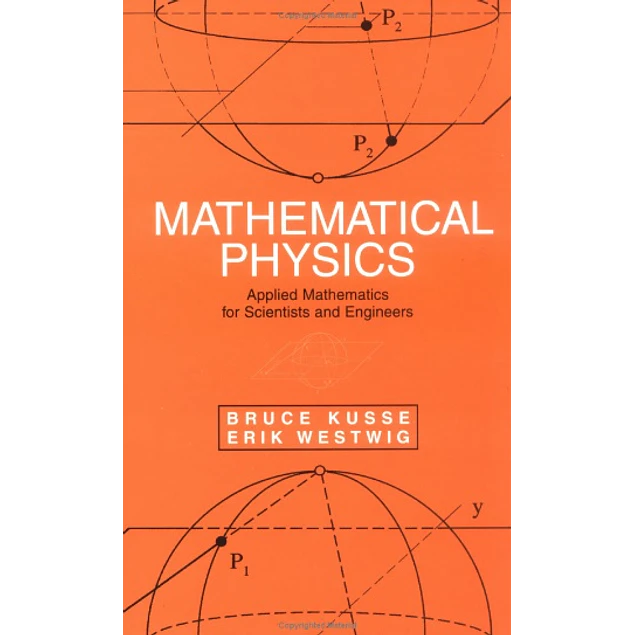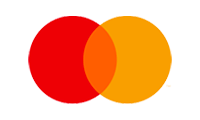## Mathematical Physics: Applied Mathematics for Scientists and Engineers

10.00\$# Mathematical Physics: Applied Mathematics for Scientists and Engineers

10.00\$

1st Edition

by Bruce R. Kusse (Author), Erik A. Westwig (Author)

What sets this volume apart from other mathematics texts is its emphasis on mathematical tools commonly used by scientists and engineers to solve real-world problems. Using a unique approach, it covers intermediate and advanced material in a manner appropriate for undergraduate students. Based on author Bruce Kusse's course at the Department of Applied and Engineering Physics at Cornell University, Mathematical Physics begins with essentials such as vector and tensor algebra, curvilinear coordinate systems, complex variables, Fourier series, Fourier and Laplace transforms, differential and integral equations, and solutions to Laplace's equations. The book moves on to explain complex topics that often fall through the cracks in undergraduate programs, including the Dirac delta-function, multivalued complex functions using branch cuts, branch points and Riemann sheets, contravariant and covariant tensors, and an introduction to group theory.

This remarkable book:
* Covers applications in all areas of engineering and the physical sciences.
* Features numerous figures and worked-out examples throughout the text.
* Presents mathematically advanced material in a readable form with few formal proofs.
* Organizes topics pedagogically in - the order they will be most easily understood.
* Provides end-of-chapter exercises.

Mathematical Physics is an excellent text for upper-level undergraduate students in physics, applied physics, physical chemistry, biophysics, and all areas of engineering. It allows physics professors to prepare students for a wide range of employment in science and engineering and makes an excellent reference for scientists and engineers in industry.

An Instructor's Manual presenting detailed solutions to all the problems in the book is available from the Wiley editorial department.

Year:
2006
Pages:
684
Language:
English
Format:
PDF
Size:
41 MB
ISBN-10:
0471154318, 3527406727
ISBN-13:
978-0471154310, 978-3527406722
##### Contact
•••••